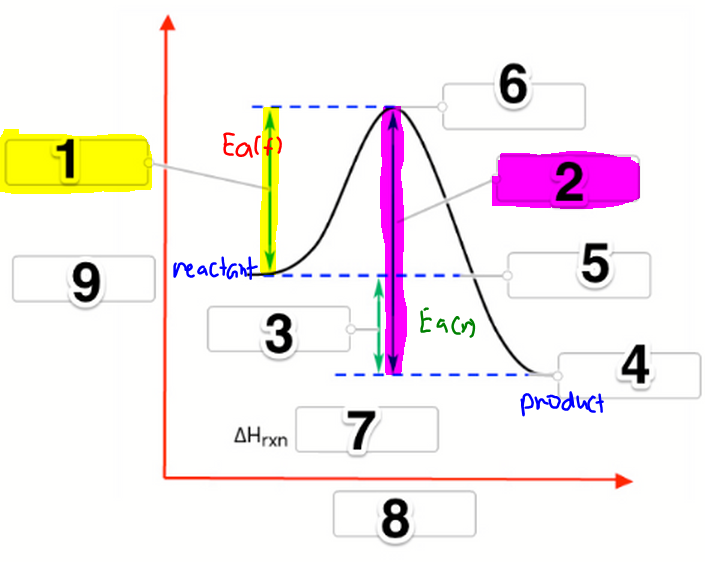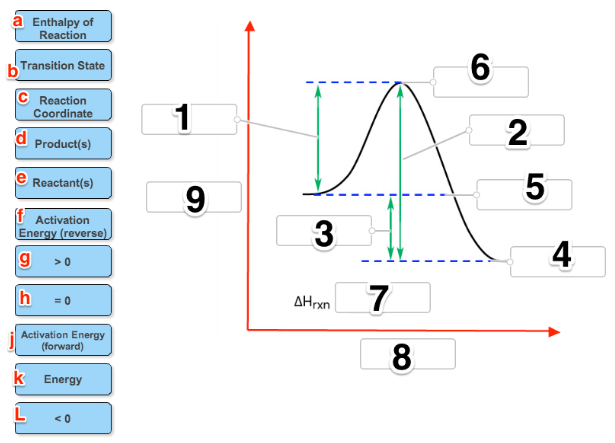# Problem: Label the following reaction coordinate diagram by matching between letters and numbers:

###### FREE Expert Solution

In this problem, we are asked to fill in the labels in the reaction coordinate diagram with the terms on the left side. So let's go through this one by one:###### Problem Details

Label the following reaction coordinate diagram by matching between letters and numbers:What scientific concept do you need to know in order to solve this problem?

Our tutors have indicated that to solve this problem you will need to apply the Energy Diagram concept. If you need more Energy Diagram practice, you can also practice Energy Diagram practice problems.

What is the difficulty of this problem?

Our tutors rated the difficulty ofLabel the following reaction coordinate diagram by matching ...as medium difficulty.

How long does this problem take to solve?

Our expert Chemistry tutor, Dasha took 4 minutes and 24 seconds to solve this problem. You can follow their steps in the video explanation above.

What professor is this problem relevant for?

Based on our data, we think this problem is relevant for Professor Ung's class at MC MARICOPA.